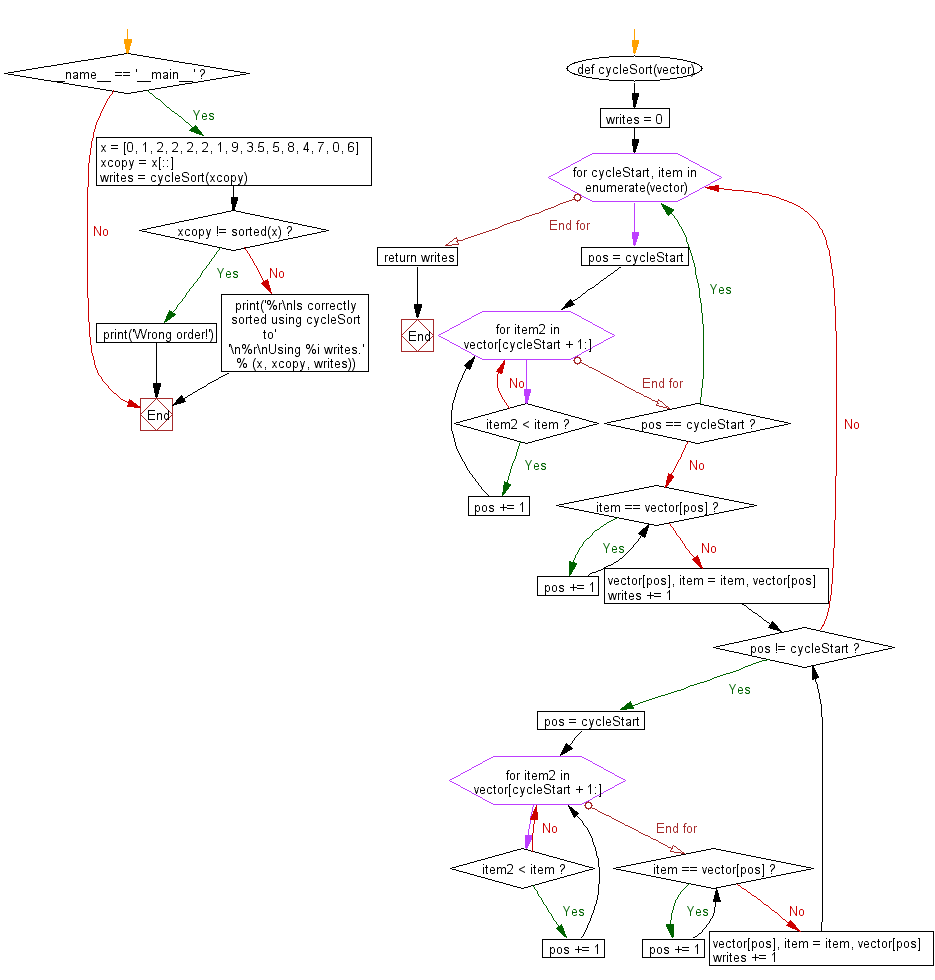﻿ Python: Sort a list of elements using Cycle sort - w3resource# Python: Sort a list of elements using Cycle sort

## Python Search and Sorting : Exercise-16 with Solution

Write a Python program to sort a list of elements using Cycle sort.
Cycle sort is an in-place, unstable sorting algorithm, a comparison sort that is theoretically optimal in terms of the total number of writes to the original array, unlike any other in-place sorting algorithm. It is based on the idea that the permutation to be sorted can be factored into cycles, which can individually be rotated to give a sorted result.

Sample Solution:

Python Code:

``````# License: https://bit.ly/2V5W81t
def cycleSort(vector):
"Sort a vector in place and return the number of writes."
writes = 0

# Loop through the vector to find cycles to rotate.
for cycleStart, item in enumerate(vector):

# Find where to put the item.
pos = cycleStart
for item2 in vector[cycleStart + 1:]:
if item2 < item:
pos += 1

# If the item is already there, this is not a cycle.
if pos == cycleStart:
continue

# Otherwise, put the item there or right after any duplicates.
while item == vector[pos]:
pos += 1
vector[pos], item = item, vector[pos]
writes += 1

# Rotate the rest of the cycle.
while pos != cycleStart:

# Find where to put the item.
pos = cycleStart
for item2 in vector[cycleStart + 1:]:
if item2 < item:
pos += 1

# Put the item there or right after any duplicates.
while item == vector[pos]:
pos += 1
vector[pos], item = item, vector[pos]
writes += 1

return writes

if __name__ == '__main__':
x = [0, 1, 2, 2, 2, 2, 1, 9, 3.5, 5, 8, 4, 7, 0, 6]
xcopy = x[::]
writes = cycleSort(xcopy)
if xcopy != sorted(x):
print('Wrong order!')
else:
print('%r\nIs correctly sorted using cycleSort to'
'\n%r\nUsing %i writes.' % (x, xcopy, writes))
```
```

Sample Output:

```[0, 1, 2, 2, 2, 2, 1, 9, 3.5, 5, 8, 4, 7, 0, 6]
Is correctly sorted using cycleSort to
[0, 0, 1, 1, 2, 2, 2, 2, 3.5, 4, 5, 6, 7, 8, 9]
Using 10 writes.
```

Flowchart:Python Code Editor:

What is the difficulty level of this exercise?

Test your Programming skills with w3resource's quiz.

﻿

## Python: Tips of the Day

Use Reversed() In for Loops:

```>>> tasks = ['laundry', 'picking up kids', 'gardening', 'cooking']